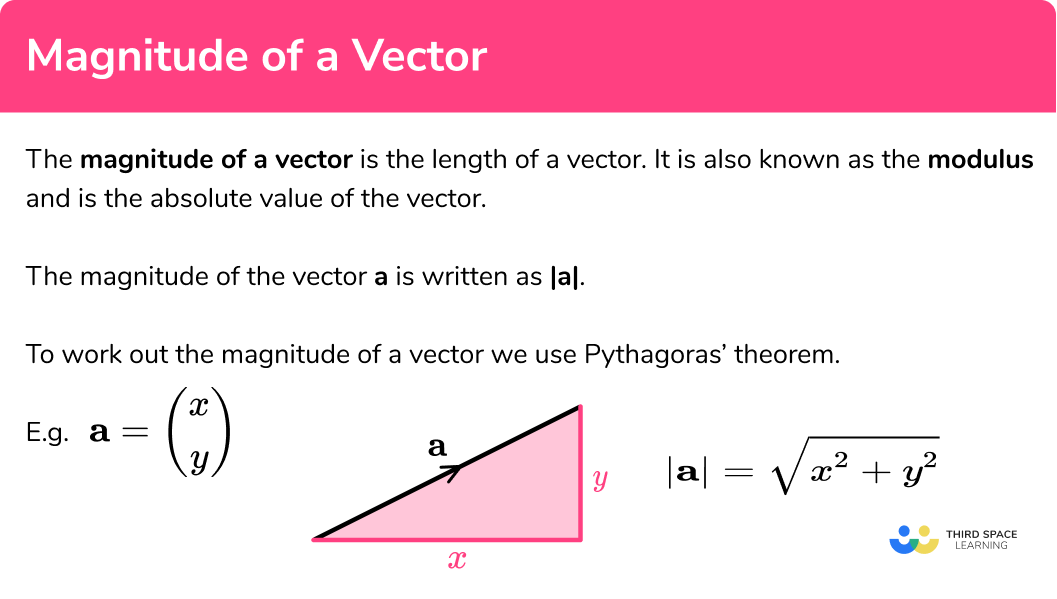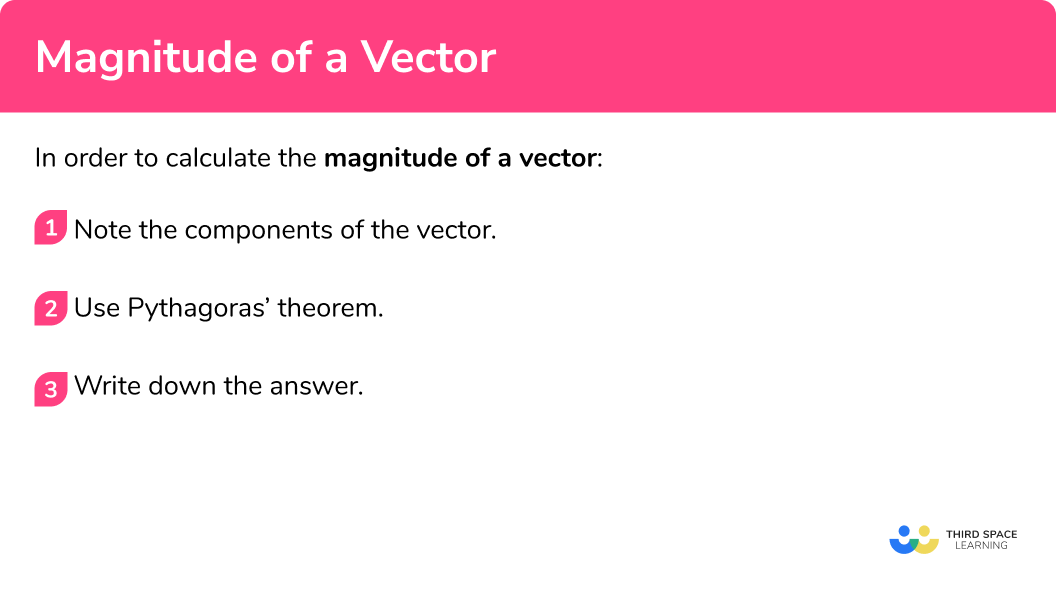GCSE Maths Geometry and Measure Vectors

Magnitude of a Vector

# Magnitude Of A Vector

Here we will learn about the magnitude of a vector, including what the magnitude of a vector is and how to calculate it.

There are also vector worksheets based on Edexcel, AQA and OCR exam questions, along with further guidance on where to go next if you’re still stuck.

## What is the magnitude of a vector?

The magnitude of a vector is the length of a vector. It is also known as the modulus or the absolute value of the vector.

The magnitude of the vector \textbf{a} is written as \lvert \textbf{a} \rvert

To work out the magnitude of a vector we use the Pythagorean theorem.

In general, we can develop a formula:

\textbf{a}= \begin{pmatrix} \; x \;\\ \; y \; \end{pmatrix}

\lvert \textbf{a} \rvert=\sqrt{x^2+y^2}

If a vector has a magnitude of 1 , it is a unit vector

E.g.

\textbf{a}= \begin{pmatrix} \; 4 \;\\ \; 3 \; \end{pmatrix}

Vector \textbf{a} has two components.

There is a horizontal component and a vertical component.  Using the initial point and the terminal point of the vector, the components of the vector make a right-angled triangle. The length of the vector is the hypotenuse of the right-angled triangle.

The magnitude of vector \textbf{a} is

\sqrt{4^2+3^2}=\sqrt{25}=5

The length of the vector is 5 .

### What is the magnitude of a vector?## How to calculate the magnitude of a vector

In order to calculate the magnitude of a vector:

1. Note the components of the vector.
2. Use Pythagoras’ theorem.

### Explain how to calculate the magnitude of a vector## Related lessons on vectors

Magnitude of a vector is part of our series of lessons to support revision on vectors. You may find it helpful to start with the main vectors lesson for a summary of what to expect, or use the step by step guides below for further detail on individual topics. Other lessons in this series include:

## Magnitude of a vector examples

### Example 1: magnitude of a vector

Find the magnitude of vector \textbf{b} , giving your answer to 1 decimal place:

\textbf{b}= \begin{pmatrix} \; 5 \;\\ \; 2 \; \end{pmatrix}

1. Note the components of the vector.

The horizontal component is x=5

The vertical component is y=2

2Use Pythagoras’ theorem.

Use the components

\sqrt{x^2+y^2}=\sqrt{5^2+2^2}=\sqrt{29}

The magnitude of the vector is

\sqrt{29}=5.3851…=5.4 \ \text{(to } 1 \text{ decimal place)}

### Example 2: magnitude of a vector

Find the magnitude of vector \textbf{v} , giving your answer to 1 decimal place:

\textbf{v}= \begin{pmatrix} \; -1 \;\\ \; 3 \; \end{pmatrix}

The horizontal component is x=-1

The vertical component is y=3

Use the components

\sqrt{x^2+y^2}=\sqrt{(-1)^2+3^2}=\sqrt{10}

The magnitude of the vector is

\sqrt{10}=3.1622…=3.2 \ \text{(to } 1 \text{ decimal place)}

### Example 3: magnitude of a vector

Find the magnitude of vector \textbf{a} , giving your answer to 1 decimal place:

\textbf{a}= \begin{pmatrix} \; -4 \;\\ \; 5 \; \end{pmatrix}

The horizontal component is x=-4

The vertical component is y=5

Use the components

\sqrt{x^2+y^2}=\sqrt{(-4)^2+5^2}=\sqrt{41}

The magnitude of the vector is

\sqrt{41}=6.4031…=6.4 \ \text{(to } 1 \text{ decimal place)}

### Example 4: magnitude of a vector

Find the magnitude of vector \textbf{b} , giving your answer to 1 decimal place:

\textbf{b}= \begin{pmatrix} \; -2 \;\\ \; -3 \; \end{pmatrix}

The horizontal component is x=-2

The vertical component is y=-3

Use the components

\sqrt{x^2+y^2}=\sqrt{(-2)^2+(-3)^2}=\sqrt{13}

The magnitude of the vector is

\sqrt{13}=3.6055…=3.6 \ \text{(to } 1 \text{ decimal place)}

### Example 5: magnitude of a vector

Find the magnitude of vector \textbf{c} , giving your answer to 1 decimal place:

\textbf{c}= \begin{pmatrix} \; -4 \;\\ \; -6 \; \end{pmatrix}

The horizontal component is x=-4

The vertical component is y=-6

Use the components

\sqrt{x^2+y^2}=\sqrt{(-4)^2+(-6)^2}=\sqrt{52}

The magnitude of the vector is

\sqrt{52}=7.2111…=7.2 \ \text{(to } 1 \text{ decimal place)}

### Example 6: magnitude of a vector

Find the magnitude of vector \textbf{v} , giving your answer to 1 decimal place:

\textbf{v}= \begin{pmatrix} \; 2 \;\\ \; -7 \; \end{pmatrix}

The horizontal component is x=2

The vertical component is y=-7

Use the components

\sqrt{x^2+y^2}=\sqrt{2^2+(-7)^2}=\sqrt{53}

The magnitude of the vector is

\sqrt{53}=7.2801…=7.3 \ \text{(to } 1 \text{ decimal place)}

### Common misconceptions

• Be careful when squaring negative values

A negative number squared gives a positive answer. If you need to square a negative number using a calculator, it is a good idea to use brackets to make sure that you get the correct answer.

### Practice magnitude of a vector questions

1. Calculate the magnitude of the vector. Give your answer to 1 decimal place.

\begin{pmatrix} \; 3 \;\\ \; 8 \; \end{pmatrix}

8.64.84.98.5The magnitude of the vector is

\sqrt{x^2+y^2}=\sqrt{3^2+8^2}=\sqrt{73}=8.544…=8.5 \ \text{to } 1 \text{ decimal place}

2. Calculate the magnitude of the vector. Give your answer to 1 decimal place.

\begin{pmatrix} \; 4 \;\\ \; 7 \; \end{pmatrix}

8.05.38.15.2The magnitude of the vector is

\sqrt{x^2+y^2}=\sqrt{4^2+7^2}=\sqrt{65}=8.062…=8.1 \ \text{to } 1 \text{ decimal place}

3. Calculate the magnitude of the vector. Give your answer to 1 decimal place.

\begin{pmatrix} \; 1 \;\\ \; -3 \; \end{pmatrix}

3.11.73.21.8The magnitude of the vector is

\sqrt{x^2+y^2}=\sqrt{1^2+(-3)^2}=\sqrt{10}=3.162…=3.2 \ \text{to } 1 \text{ decimal place}

4. Calculate the magnitude of the vector. Give your answer to 1 decimal place.

\begin{pmatrix} \; 2 \;\\ \; -4 \; \end{pmatrix}

4.54.45.15.2The magnitude of the vector is

\sqrt{x^2+y^2}=\sqrt{2^2+(-4)^2}=\sqrt{20}=4.472…=4.5 \ \text{to } 1 \text{ decimal place}

5. Calculate the magnitude of the vector. Give your answer to 1 decimal place.

\begin{pmatrix} \; -3 \;\\ \; 2 \; \end{pmatrix}

3.73.62.42.5The magnitude of the vector is

\sqrt{x^2+y^2}=\sqrt{(-3)^2+2^2}=\sqrt{13}=3.605…=3.6 \ \text{to } 1 \text{ decimal place}

6. Calculate the magnitude of the vector. Give your answer to 1 decimal place.

\begin{pmatrix} \; -1 \;\\ \; -4 \; \end{pmatrix}

4.25.14.15.2The magnitude of the vector is

\sqrt{x^2+y^2}=\sqrt{(-1)^2+(-4)^2}=\sqrt{17}=4.123…=4.1 \ \text{to } 1 \text{ decimal place}

### Magnitude of a vector GCSE questions

1. What is the magnitude of this vector?

\begin{pmatrix} \; -4 \;\\ \; 0 \; \end{pmatrix}

(2 marks)

\sqrt{(-4)^{2}+0^{2}}

(For using Pythagoras’ theorem)

(1)

=\sqrt{16}=4

(1)

2. Here is a column vector.

\textbf{a}= \begin{pmatrix} \; 7 \;\\ \; 3 \; \end{pmatrix}

Calculate the magnitude of the vector \textbf{a} .

(2 marks)

\sqrt{7^2+3^2}

(For using Pythagoras’ theorem)

(1)

=\sqrt{58}=7.61577…=7.62 \ \text{to } 3sf

(1)

3. Here is a column vector.

\textbf{b}= \begin{pmatrix} \; -4 \;\\ \; 9 \; \end{pmatrix}

Calculate the magnitude of the vector \textbf{b} .

(2 marks)

\sqrt{(-4)^2+9^2}

(For using Pythagoras’ theorem)

(1)

=\sqrt{97}=9.84885…=9.85 \ \text{to } 3sf

(1)

## Learning checklist

You have now learned how to:

• Calculate the magnitude of a vector using Pythagoras’ theorem

## Did you know?

Vectors are studied more beyond GCSE.  If a vector has a magnitude of 1 , it is a unit vector.  The magnitude of a vector  can be used to calculate the dot product of two vectors.  It is also possible to calculate the cross product of two vectors.

## Still stuck?

Prepare your KS4 students for maths GCSEs success with Third Space Learning. Weekly online one to one GCSE maths revision lessons delivered by expert maths tutors.

Find out more about our GCSE maths tuition programme.